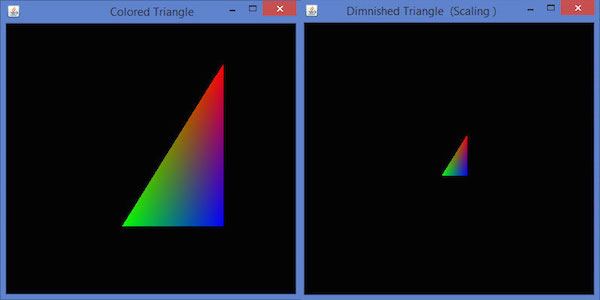# JOGL - Scaling

This chapter teaches you how to scale an object ie., increase or decrease the size of an object using JOGL.

Scaling an object is done by using the glScalef(float x, float y, float z) method of GLMatrixFunc interface. This method accepts three floating point parameters, using which we specify the scale factors along the x, y, and z axes respectively.

For example, in the following program, a triangle is diminished to 50%. Here, the value 50 is passed as parameter along all the axes.

Let us go through the program to scale a triangle −

```import javax.media.opengl.GL2;
import javax.media.opengl.GLAutoDrawable;
import javax.media.opengl.GLCapabilities;
import javax.media.opengl.GLEventListener;
import javax.media.opengl.GLProfile;
import javax.media.opengl.awt.GLCanvas;

import javax.swing.JFrame;

public class Scaling implements GLEventListener {

@Override

public void display( GLAutoDrawable drawable ) {

final GL2 gl = drawable.getGL().getGL2();
gl.glScalef( 0.50f,0.25f,0.50f );
gl.glBegin( GL2.GL_TRIANGLES );

// Drawing Using Triangles
gl.glColor3f( 1.0f, 0.0f, 0.0f );   // Red
gl.glVertex3f( 0.5f,0.7f,0.0f );    // Top

gl.glColor3f( 0.0f,1.0f,0.0f );     // blue
gl.glVertex3f( -0.2f,-0.50f,0.0f ); // Bottom Left

gl.glColor3f( 0.0f,0.0f,1.0f );     // green
gl.glVertex3f( 0.5f,-0.5f,0.0f );   // Bottom Right

gl.glEnd();
}

@Override
public void dispose( GLAutoDrawable arg0 ) {
//method body
}

@Override
public void init( GLAutoDrawable arg0 ) {
// method body
}

@Override
public void reshape( GLAutoDrawable arg0, int arg1, int arg2, int arg3, int arg4 ) {
// method body
}

public static void main( String[] args ) {

//getting the capabilities object of GL2 profile
final GLProfile profile = GLProfile.get( GLProfile.GL2 );
GLCapabilities capabilities = new GLCapabilities(profile);

// The canvas
final GLCanvas glcanvas = new GLCanvas( capabilities );
Scaling scaling = new Scaling();
glcanvas.setSize( 400, 400 );

//creating frame
final JFrame frame  = new JFrame (" Dimnished Triangle (Scaling )");

frame.setSize(frame.getContentPane().getPreferredSize());
frame.setVisible(true);

} //end of main

} //end of classimport javax.media.opengl.GL2;
```

On compiling and executing the above program, we get the following output. Here, you can observe a diminished triangle as compared to the original triangle produced by TriangleColor.java −